Year 10 Interactive Maths - Second Edition

## Scatterplots

A scatterplot is a graph that represents bivariate data as points on a two-dimensional Cartesian plane.

#### Example 12

The following set of data values was observed for the height h (in cm) and weight w (in kg) of nine Year 10 students.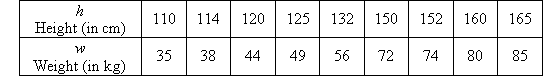Plot a scatterplot for this set of data.

##### Solution:

The scatterplot is obtained by plotting w against h, as shown below.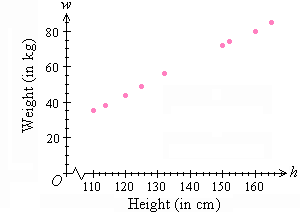We use the scatterplot to look for patterns that might indicate that the variables are related.  Then, if the variables are related, we can visualise what kind of line (or curve), or equation, describes the relationship.

## Association (or Relationship)

Association (or relationship) between two variables will be described as strong, weak or none; and the direction of the association may be positive, negative or none.

In the previous example, w increases as h increases. We say that a strong positive association exists between the variables h and w.

Consider the following scatterplot: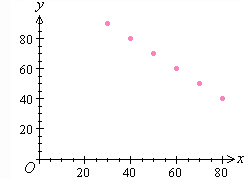It is clear from the scatterplot that y decreases as x increases. We say that a strong negative association exists between the variables x and y.

Consider the following scatterplot: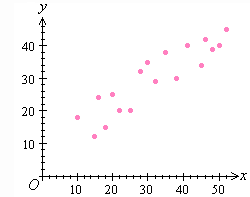We observe that y increases as x increases, and the points do not lie on a straight line. We say that a weak positive association exists between the variables x and y.

Consider the following scatterplot: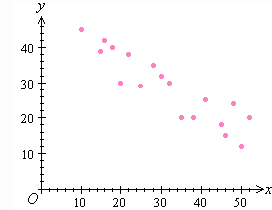We observe that y decreases as x increases, and the points do not lie on a straight line.  We say that a weak negative association exists between the variables x and y.

Consider the following scatterplot: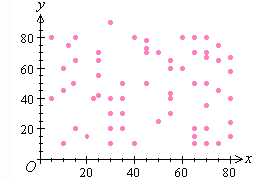It is clear from the scatterplot that as x increases, there is no apparent effect on the y.  In such a case, we say that no association exists between the variables x and y.

Consider the following scatterplot: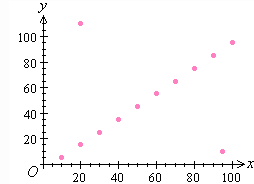If a data value does not fit the trend of the data, then it is said to be an outlier.  In the above scatterplot, it is easy to identify the outliers. There are two outliers in the set of data values.